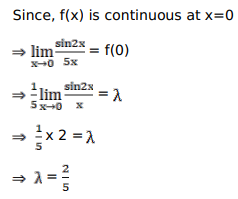# Find the value of k for whichQuestion:

Find the value of $k$ for which

$f(x)=\left\{\begin{array}{c}\frac{\sin 2 x}{5 x}, \text { when } x \neq 0 ; \\ \lambda, \text { when } x=0\end{array}\right.$ is continuous at $x=0$

Solution: